Questions from the forum
 Q: I'd like to have a countdown timer that shuts down the game after 10 minutes of play. Can you help? A: There you go.   var time_left = 600; // allow the player to run the game for 600 seconds (10 minutes) var minutes_left = 0; var seconds_left = 0;   STRING* time_str = "#30"; STRING* temp_str = "#10";   TEXT* time_txt = {        pos_x = 20;        pos_y = 20;        string(time_str);        flags = SHOW; }   function countdown_startup() {        while (time_left >= 0)        {                minutes_left = integer(time_left / 60);                seconds_left = time_left - minutes_left * 60;                str_cpy(time_str, "Time left to play: ");                str_for_num(temp_str, minutes_left);                str_cat(time_str, temp_str);                str_cat(time_str, ":");                str_for_num(temp_str, seconds_left);                if (seconds_left < 10)                        str_cat(time_str, "0");                str_cat(time_str, temp_str);                time_left -= 1;                wait (-1);        }        sys_exit(NULL); }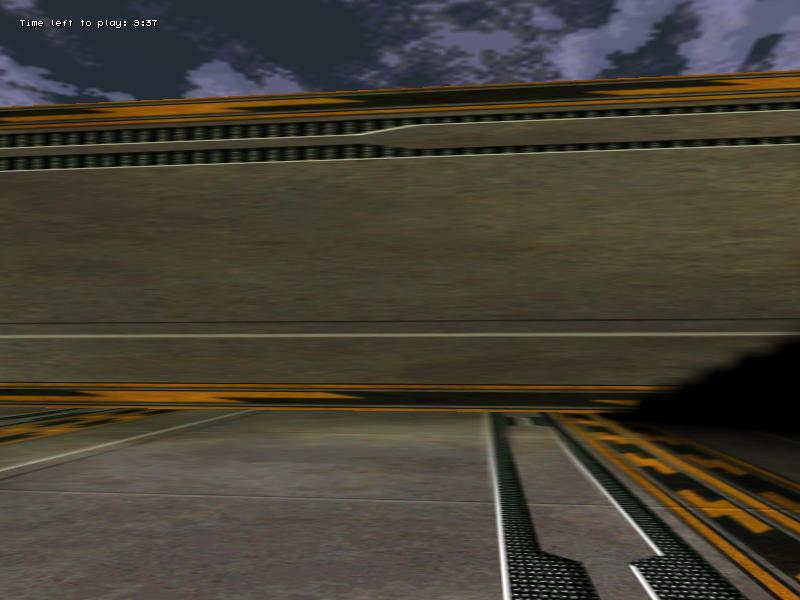Q: I'd like to adjust the frame rate through a slider on a panel, thus increasing and decreasing the navigation speed in my level. A: You don't want to do that - it will make your game run choppy at low frame rates. Use time_factor instead - here's an example that moves an entity on a path and allows you to control its speed.   var entity_speed = 3; var movement_enabled = 0; var dist_to_node; var current_node = 1; var angle_difference = 0;   VECTOR temp_angle; VECTOR pos_node; // stores the position of the node   BMAP* slider_tga = "slider.tga"; BMAP* pointer_tga = "pointer.tga";   PANEL* speed_pan = {        bmap = "speed_panel.tga";        pos_x = 0;        pos_y = 0;        hslider(16, 24, 298, slider_tga, 0, 10, time_factor);        flags = SHOW; }   function mouse_startup() {         mouse_mode = 2;        mouse_map = pointer_tga;        while (1)        {                 vec_set(mouse_pos, mouse_cursor);                wait(1);        } }   function move_target() {        while(1)        {                if(movement_enabled)                {                        entity_speed = minv(5, entity_speed + 0.5 * time_step);                        c_move(my, vector(entity_speed * time_step, 0, 0), nullvector, IGNORE_PASSABLE | GLIDE);                        vec_to_angle (my.pan, vec_diff (temp_angle, pos_node, my.x));                }                wait(1);        } }   action move_on_path() // attach this action to a model {               move_target();        result = path_scan(me, my.x, my.pan, vector(360, 180, 1000));        if (result) {movement_enabled = 1;}        path_getnode (my, 1, pos_node, NULL);        vec_to_angle (my.pan, vec_diff (temp_angle, pos_node, my.x)); // rotate towards the node        while(1)        {                dist_to_node = vec_dist(my.x, pos_node);                if(dist_to_node < 50) // close to the node?                {                        current_node = path_nextnode(my, current_node, 1);                        if (!current_node) {current_node = 1;} // reached the end of the path? Then start over!                        path_getnode (my, current_node, pos_node, NULL);                }                wait(1);        } }     Q: Is there any chance to have a door scan its surroundings and slide horizontally when the player approaches it? A: Sure, take a look.   var slide_once = 0;   action sliding_door() {        while (!player) {wait (1);}        while (1)          {                  // the door scans on a range of 300 quants, trying to detect the "player" entity                if ((c_scan(my.x, my.pan, vector(360, 180, 300), IGNORE_ME) > 0) && (you == player))                {                        slide_once += 1;                        if (slide_once == 1) // do this only once                        {                                // this door slides along the x axis, feel free to use the y and z axis if they serve you better                                my.skill90 = my.x;                                while (my.x < my.skill90 + 100) // the door slides 100 quants along the x axis                                {                                        my.x += 3 * time_step; // 3 gives the sliding speed                                                wait (1);                                }                        }                }                else // the player has moved away from the door here                {                        slide_once = 0; // reset slide_once                        while (my.x > my.skill90) // let's move the door back to its initial position                        {                                my.x -= 3 * time_step; // 3 gives the sliding speed                                        wait (1);                        }                        my.x = my.skill90; // make sure that the end x position is the same with the initial x position                }                  wait (1);        } }   action players_code() // simple player code snippet {        var movement_speed = 10; // movement speed        VECTOR temp;        player = my; // I'm the player        while (1)        {                my.pan -= 7 * mouse_force.x * time_step;                camera.x = my.x;                camera.y = my.y;                camera.z = my.z + 50 + 1.1 * sin(my.skill44); // play with 50 and 1.1                camera.pan = my.pan;                camera.tilt += 5 * mouse_force.y * time_step;                vec_set (temp.x, my.x); // trace 10,000 quants below the player                temp.z -= 10000;                temp.z = -c_trace (my.x, temp.x, IGNORE_ME | IGNORE_PASSABLE | USE_BOX) - 2; // play with 2                temp.x = movement_speed * (key_w - key_s) * time_step;                temp.y = movement_speed * (key_a - key_d) * 0.6 * time_step;                c_move (my, temp.x, nullvector, IGNORE_PASSABLE | GLIDE);                wait (1);        } }     Q: I started to work at a racing game and I'd like to have a car selection screen, where the player uses two buttons to go through all the available car models. A: Create a tiny room, place your car inside it and then use the code below.   var car_type = 1; // showing the first car model at game start   BMAP* arrowleft_pcx = "arrowleft.pcx"; BMAP* arrowright_pcx = "arrowright.pcx"; BMAP* pointer_tga = "pointer.tga";   STRING* tinylevel_wmb = "test.wmb";   ENTITY* car;   function cars_left(); function cars_right(); function set_current_car();   PANEL* leftclick_pan = {        bmap = "arrowleft.pcx";        pos_x = 0;        pos_y = 0;        layer = 15;        on_click = cars_left;        flags = SHOW; }   PANEL* rightclick_pan = {        bmap = "arrowright.pcx";        pos_x = 750;        pos_y = 0;        layer = 15;        on_click = cars_right;        flags = SHOW; }   void main() {        fps_max = 70;        video_mode = 7; // run in 800x600 pixels        video_depth = 32; // 32 bit mode        video_screen = 1; // start in full screen mode        level_load (tinylevel_wmb);        while (!car) {wait (1);} // wait until the car model is loaded        // choose a proper camera position that allows you to see the cars perfectly        vec_set (camera.x, vector(1000, 300, 200));        // and maybe set the camera pan, tilt and roll angles as well        vec_set (camera.pan, vector(100, -20, 5)); }   function mouse_startup() {         mouse_mode = 2;        mouse_map = pointer_tga;        while (1)        {                 vec_set(mouse_pos, mouse_cursor);                wait(1);        } }   function cars_left() {        car_type -= 1;        car_type = maxv(1, car_type);        set_current_car(); }   function cars_right() {        car_type += 1;        car_type = minv(3, car_type);        set_current_car(); }   function set_current_car() {        if(car_type == 1)                  ent_morph(car, "car1.mdl");        if(car_type == 2)                ent_morph(car, "car2.mdl");        if(car_type == 3)                ent_morph(car, "car3.mdl");   // add as many cars as you want here         }       // attach this action to the car1.mdl model (the default car) action my_cars() {        car = my;        while (1)        {                my.pan += 3 * time_step;                wait (1);        } }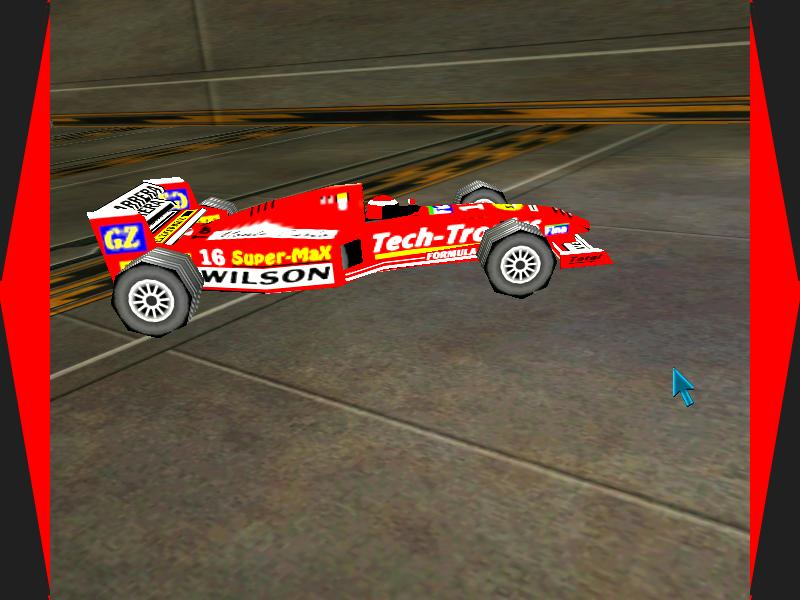Q: Did anyone use the string1 and string2 strings from within Wed? I'd like to see a simple example, if possible. A: Here's an example that shows the content of string1 / string2 when an entity is clicked with the left / right mouse buttons.   BMAP* pointer_tga = "pointer.tga";   STRING* info_str = "#32";   function mouse_startup() {         mouse_mode = 2;        mouse_map = pointer_tga;        while (1)        {                 vec_set(mouse_pos, mouse_cursor);                wait(1);        } }   TEXT* info_txt = {        pos_x = 50;        pos_y = 20;        string (info_str);        flags = SHOW; }   function strings_startup() {        while (1)        {                if (mouse_ent)                {                        if (mouse_left)                                str_cpy(info_str, mouse_ent.string1); // put your text in entity's string1 in Wed                        if (mouse_right)                                str_cpy(info_str, mouse_ent.string2); // put your text in entity's string1 in Wed                                                        set(info_txt, SHOW);                }                else                {                        reset(info_txt, SHOW);                }                wait (1);        } }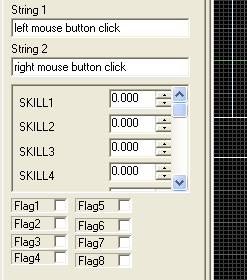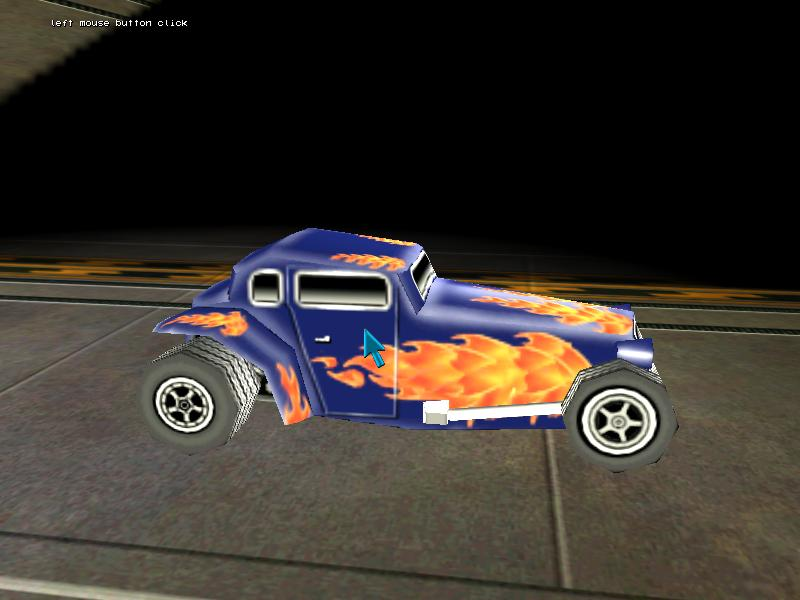Q: I'm looking for the easiest way to make drop shadows, that you can see in various games under the characters. A: Here's a simple, and yet fully functional example that allows you to play with several types of shadows.   #include #include #include // contains the stencil_blur() function   STRING* test_wmb = "test.wmb";   function main() {        camera.ambient = 70;        fps_max = 70;        video_mode = 7; // run in 800x600 pixels        video_depth = 32; // 32 bit mode        video_screen = 1; // start in full screen mode        level_load (test_wmb);        wait (2);          ent_createlayer("skycube+6.tga", SKY | CUBE | SHOW, 1);                shadow_stencil = 4; // use values in the 0...4 range        shadow_lod = 2; // use the second LOD stage for stencil shadows        stencil_blur(1); // activate blurred shadows        (included in mtlView.c, look better) }   action shadow_model() // simple action that rotates this model in a circle {        set(my, SHADOW);        while (1)        {                c_move (my, vector(5 * time_step, 0, 0), nullvector, IGNORE_PASSABLE | GLIDE);                  my.pan += 2 * time_step; // 2 sets the radius of the circle                  wait (1);        } }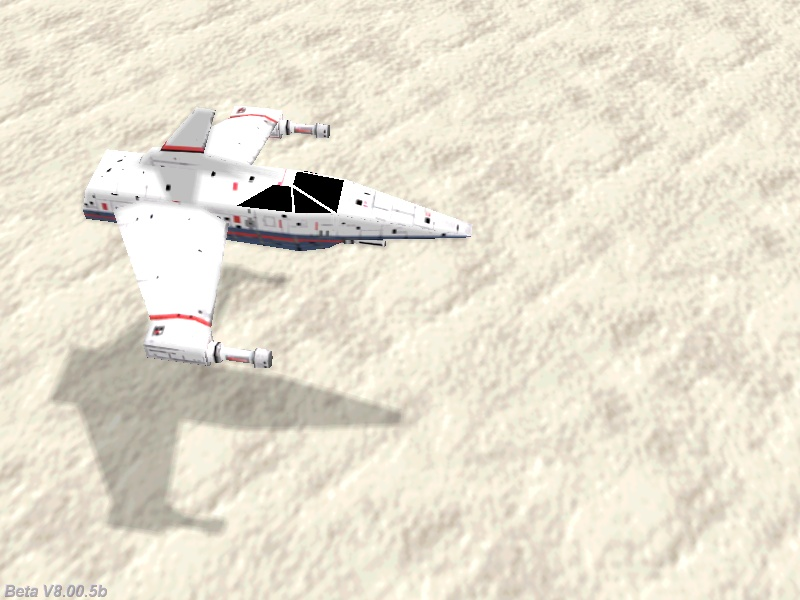Q: I don't know if  this is covered anywhere, but how do I make the number pad keys (2, 4, 6, 8) control player's movement? A: Here's a fully functional example.   action players_code() // attach this action to your player {                var movement_speed = 20;        VECTOR temp;        set (my, INVISIBLE);        player = my;        while (1)        {                my.pan -= 7 * mouse_force.x * time_step;                camera.x = my.x;                camera.y = my.y;                camera.z = my.z + 50 + 1.1 * sin(my.skill44);                camera.pan = my.pan;                camera.tilt += 5 * mouse_force.y * time_step;                vec_set (temp.x, my.x);                temp.z -= 10000;                temp.z = -c_trace (my.x, temp.x, IGNORE_ME | IGNORE_PASSABLE | USE_BOX) - 2;                temp.x = movement_speed * (key_pressed(72) - key_pressed(80)) * time_step;                temp.y = movement_speed * (key_pressed(75) - key_pressed(77)) * 0.6 * time_step;                c_move (my, temp.x, nullvector, IGNORE_PASSABLE | GLIDE);                wait (1);        } }     Q: How can I create a health bar for my player character? A: There you go.   var players_health;   PANEL* health_pan = {        bmap = "redbar.pcx"; // a bitmap with x = 2, y = 12 pixels        pos_x = -10;        pos_y = 0;        layer = 10;        flags = SHOW; }   function health_startup() {        while (1)        {                health_pan.scale_x = maxv(0.001, players_health * 3.5); // 3.5 = experimental value                wait (1);        } }                                 action players_code() // attach this action to your player {                var movement_speed = 20;        VECTOR temp;        set (my, INVISIBLE);        player = my;        players_health = 100; // the player starts with 100 health points        while (players_health > 0)        {                my.pan -= 7 * mouse_force.x * time_step;                camera.x = my.x;                camera.y = my.y;                camera.z = my.z + 50 + 1.1 * sin(my.skill44);                camera.pan = my.pan;                camera.tilt += 5 * mouse_force.y * time_step;                vec_set (temp.x, my.x);                temp.z -= 10000;                temp.z = -c_trace (my.x, temp.x, IGNORE_ME | IGNORE_PASSABLE | USE_BOX) - 2;                temp.x = movement_speed * (key_w - key_s) * time_step;                temp.y = movement_speed * (key_a - key_d) * 0.6 * time_step;                c_move (my, temp.x, nullvector, GLIDE);                wait (1);        }        // the player is dead here        camera.z -= 20;        camera.roll = -35; }   action health_taker() {        while (!player) {wait (1);}        while (1)        {                if (vec_dist(player.x, my.x) < 200)                        players_health -= 2 * time_step;                wait (1);        } }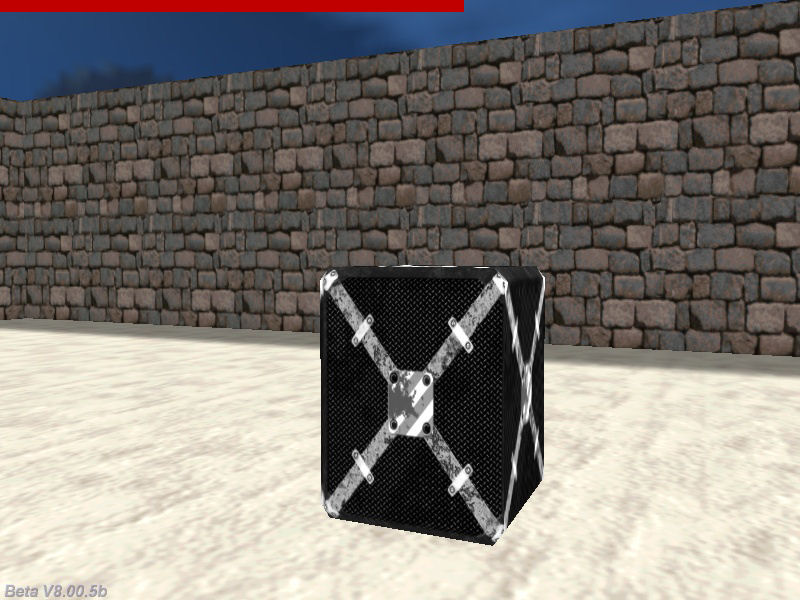Q: I'd like to have a camera that can rotate around the player whenever I want it to. Player's movement would be done using the WSAD keys and the camera rotation would be done using the OP keys. A: There you go.   function camera_startup() {        var orbit_radius = 200; // use your own value here        var orbit_speed = 4; // and here        var temp_angle;        VECTOR orbit_center, temp;        while (!player) {wait (1);}        vec_set (orbit_center.x, player.x); // store the orbit center position        while(1)        {                camera.x = orbit_center.x + sin(temp_angle) * orbit_radius;                camera.y = orbit_center.y + cos(temp_angle) * orbit_radius;                camera.z = orbit_center.z + 50; // play with 50                camera.tilt = -20; // play with -20                // use the "O" (not zero!) and "P" keys to rotate the camera around the player                temp_angle += 1 * (key_o - key_p) * orbit_speed * time_step; // 1 gives the camera rotation speed                vec_set(temp, player.x);                vec_sub(temp, camera.x);                vec_to_angle(camera.pan, temp); // make the camera look at the player all the time                wait(1);        } }   action players_code() // attach this action to your player {                var movement_speed = 20;        VECTOR temp;        player = my;        while (1)        {                my.pan -= 7 * mouse_force.x * time_step;                vec_set (temp.x, my.x);                temp.z -= 10000;                temp.z = -c_trace (my.x, temp.x, IGNORE_ME | IGNORE_PASSABLE | USE_BOX) + 20; // 20 gives the distance to ground                temp.x = movement_speed * (key_w - key_s) * time_step;                temp.y = movement_speed * (key_a - key_d) * 0.6 * time_step;                c_move (my, temp.x, nullvector, IGNORE_PASSABLE | GLIDE);                wait (1);        } }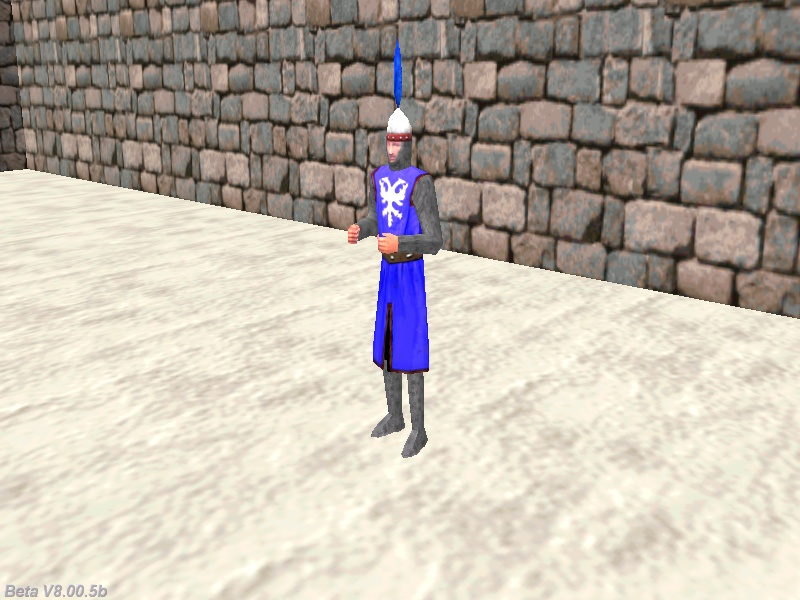Q: Is there a way to keep a particle under a moving entity? I have fire that pushes out the bottom of my entity, but when the entity moves, the particles stay were they were created, leaving a trail of fire behind. A: The easiest solution is to create particles with a very short lifespan value - here's a fully functional example.   BMAP* fire_tga = "fire.tga";   function fade_jet(PARTICLE *p) {        p.alpha -= 40 * time_step; // fade out the fire particles, play with this value        if (p.alpha < 0)                p.lifespan = 0; }   function jet_effect(PARTICLE *p) {        p->vel_x = 1 - random(2);        p->vel_y = 1 - random(2);        p->vel_z = 1 + random(1);        p.lifespan = 10; // play with this value        p.alpha = 25 + random(50);        p.bmap = fire_tga;        p.size = 25; // gives the size of the fire particles        p.flags |= (BRIGHT | MOVE);        p.event = fade_jet; }   action my_player() // dummy player action {        VECTOR jet_offset;        while (1)        {                // put your own player code here                // the example below simply makes the player rotate in a circle                c_move (my, vector(20 * time_step, 0, 0), nullvector, IGNORE_PASSABLE | GLIDE);                my.pan += 2 * time_step; // 2 sets the radius of the circle                              // place the particle jet at the proper position, play with the offset values below             vec_set(jet_offset.x, vector(-100, -10, -20));                vec_rotate(jet_offset.x, my.pan);              vec_add(jet_offset.x, my.x);             effect(jet_effect, 1, jet_offset.x, nullvector); // generate a jet particles per frame                wait (1);        } }